# A Brief Critique of Proportionality

## Introduction

We recognize that this package uses concepts that are not necessarily intuitive. As such, we offer a brief critique of proportionality analysis. Although the user may feel eager to start here, we strongly recommend first reading the companion vignette, “An Introduction to Proportionality”.

## Sample data

To facilitate discussion, we simulate count data for 5 features (e.g., genes) labeled “a”, “b”, “c”, “d”, and “e”, as measured across 100 subjects.

library(propr)
N <- 100
a <- seq(from = 5, to = 15, length.out = N)
b <- a * rnorm(N, mean = 1, sd = 0.1)
c <- rnorm(N, mean = 10)
d <- rnorm(N, mean = 10)
e <- rep(10, N)
X <- data.frame(a, b, c, d, e)

Let us assume that these data $$X$$ represent absolute abundance counts (i.e., not relative data). We can build a relative dataset, $$Y$$, by constraining and perturbing $$X$$:

Y <- X / rowSums(X) * abs(rnorm(N))

We can check that the new feature vectors do in fact contain relative quantities. For example, the ratio of the second feature to the first is the same for both the absolute and relative datasets.

all(round(X[, 2] / X[, 1] - Y[, 2] / Y[, 1], 5) == 0)
##  TRUE

## Spurious correlation

Next, we compare pairwise scatterplots for the absolute count data and the corresponding relative count data. We see quickly how these relative data suggest a spurious correlation: although genes “c” and “d” do not correlate with one another absolutely, their relative quantities do.

pairs(X) # absolute data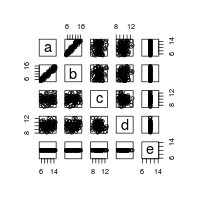pairs(Y) # relative data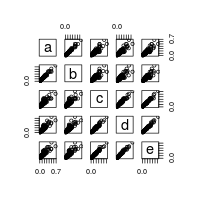Spurious correlation is evident by the correlation coefficients too.

suppressWarnings(cor(X)) # absolute correlation
##            a          b           c           d  e
## a 1.00000000 0.96236158  0.05876654  0.09772588 NA
## b 0.96236158 1.00000000  0.07813953  0.11540416 NA
## c 0.05876654 0.07813953  1.00000000 -0.17684588 NA
## d 0.09772588 0.11540416 -0.17684588  1.00000000 NA
## e         NA         NA          NA          NA  1
cor(Y) # relative correlation
##           a         b         c         d         e
## a 1.0000000 0.9870765 0.8838822 0.8971704 0.8885508
## b 0.9870765 1.0000000 0.8525697 0.8677007 0.8593081
## c 0.8838822 0.8525697 1.0000000 0.9755464 0.9850897
## d 0.8971704 0.8677007 0.9755464 1.0000000 0.9895088
## e 0.8885508 0.8593081 0.9850897 0.9895088 1.0000000

## An in-depth look at VLR

In contrast, the variance of the log-ratios (VLR), defined as the variance of the logarithm of the ratio of two feature vectors, offers a measure of dependence that (a) does not change with respect to the nature of the data (i.e., absolute or relative), and (b) does not change with respect to the number of features included in the computation. As such, the VLR, constituting the numerator portion of the $$\phi$$ metric and a portion of the $$\rho$$ metric as well, is considered sub-compositionally coherent. Yet, while VLR yields valid results for compositional data, it lacks a meaningful scale.

propr:::proprVLR(Y[, 1:4]) # relative VLR
##             a           b          c          d
## a 0.000000000 0.008307997 0.10684573 0.10215278
## b 0.008307997 0.000000000 0.12432295 0.12006896
## c 0.106845728 0.124322946 0.00000000 0.02376685
## d 0.102152784 0.120068957 0.02376685 0.00000000
propr:::proprVLR(X) # absolute VLR
##             a           b          c           d           e
## a 0.000000000 0.008307997 0.10684573 0.102152784 0.097960496
## b 0.008307997 0.000000000 0.12432295 0.120068957 0.116923511
## c 0.106845728 0.124322946 0.00000000 0.023766852 0.011702121
## d 0.102152784 0.120068957 0.02376685 0.000000000 0.008715276
## e 0.097960496 0.116923511 0.01170212 0.008715276 0.000000000

## An in-depth look at clr

In the calculation of proportionality, we adjust the arbitrarily large VLR by the variance of its individual constituents. To do this, we need to place samples on a comparable scale. Log-ratio transformation, such as the centered log-ratio (clr) transformation, shifts the data onto a “standardized” scale that allows us to compare differences in the VLR-matrix.

In the next figures, we compare pairwise scatterplots for the clr-transformed absolute count data and the corresponding clr-transformed relative count data. While equivalent, we see a relationship between “c” and “d” that should not exist based on what we know from the non-transformed absolute count data. This demonstrates that, although the clr-transformation helps us compare values across samples, it does not rescue information lost by making absolute data relative.

pairs(propr:::proprCLR(Y[, 1:4])) # relative clr-transformation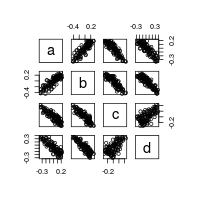pairs(propr:::proprCLR(X)) # absolute clr-transformation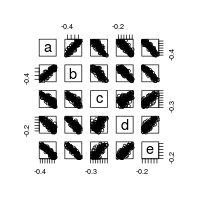Proportionality is a compromise between the advantages of VLR and the disadvantages of clr to establish a measure of dependence that is robust yet interpretable. Note, however, that because of the division of VLR by the variance of the clr-transformed data, proportionality is not sub-compositionally coherent. As such, spurious proportionality is possible when the clr does not adequately approximate the absolute data.

perb(Y[, 1:4])@matrix # relative proportionality with clr
##            a          b          c          d
## a  1.0000000  0.8537799 -0.8621586 -0.8525869
## b  0.8537799  1.0000000 -0.8772614 -0.8764010
## c -0.8621586 -0.8772614  1.0000000  0.6317950
## d -0.8525869 -0.8764010  0.6317950  1.0000000
perb(X)@matrix # absolute proportionality with clr
##            a          b          c          d          e
## a  1.0000000  0.8952555 -0.8195923 -0.8133789 -0.8676093
## b  0.8952555  1.0000000 -0.7864834 -0.7866308 -0.8464436
## c -0.8195923 -0.7864834  1.0000000  0.4900580  0.7261155
## d -0.8133789 -0.7866308  0.4900580  1.0000000  0.7839530
## e -0.8676093 -0.8464436  0.7261155  0.7839530  1.0000000

## An in-depth look at alr

Unlike the clr which adjusts each subject vector by the geometric mean of that vector, the additive log-ratio (alr) adjusts each subject vector by the value of one its own components, chosen as a reference. If we select as a reference some feature $$D$$ with an a priori known fixed absolute count across all subjects, we can effectively “back-calculate” absolute data from relative data. When initially crafting the data $$X$$, we included “e” as this fixed value.

The following figures compare pairwise scatterplots for alr-transformed relative count data (i.e., $$alr(Y)$$ with “e” as the reference) and the corresponding absolute count data. We see here how alr-transformation eliminates the spurious correlation between “c” and “d”.

pairs(propr:::proprALR(Y, ivar = 5)) # relative alr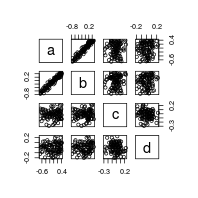pairs(X[, 1:4]) # absolute data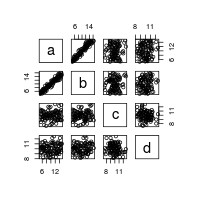Again, this gets reflected in the results of perb when we select “e” as the reference.

perb(Y, ivar = 5)@matrix # relative proportionality with alr
##            a          b           c           d e
## a 1.00000000 0.96133729  0.02568686  0.04239939 0
## b 0.96133729 1.00000000  0.03345122  0.04433209 0
## c 0.02568686 0.03345122  1.00000000 -0.16404907 0
## d 0.04239939 0.04433209 -0.16404907  1.00000000 0
## e 0.00000000 0.00000000  0.00000000  0.00000000 1

Now, let us assume these same data, $$X$$, actually measure relative counts. In other words, $$X$$ is already relative and we do not know the real quantities which correspond to $$X$$ absolutely. Well, if we knew that “a” represented a known fixed quantity, we could use alr-transformation again to “back-calculate” the absolute abundances. In this case, we will see that “c”, “d”, and “e” actually do have proportional expression under these conditions. Although the measured quantity of “c”, “d”, and “e” do not change considerably across subjects, the measured quantity of the known fixed feature does change. As such. whenever “a” increases while “c”, “d”, and “e” remains the same, the latter three features have actually decreased. Since they all decreased together, they act as a highly proportional module.

pairs(propr:::proprALR(X, ivar = 1)) # new relative alr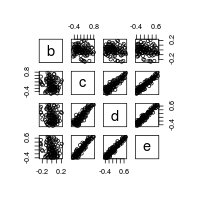Again, this gets reflected in the results of perb when we select “a” as the reference.

perb(X, ivar = 1)@matrix # new relative proportionality with alr
##   a           b           c           d          e
## a 1  0.00000000  0.00000000  0.00000000  0.0000000
## b 0  1.00000000 -0.07962592 -0.08698269 -0.1002651
## c 0 -0.07962592  1.00000000  0.88628220  0.9428625
## d 0 -0.08698269  0.88628220  1.00000000  0.9564483
## e 0 -0.10026507  0.94286248  0.95644829  1.0000000

We see here that, unlike clr-transformed proportionality metrics, the alr-transformed metric $$\rho$$ is sub-compositionally coherent and yields identical results regardless of the nature of the data explored. Of course, this assumes that one knows the identity of a feature fixed across all subjects. Although, if a reference were known, one might consider “back-calculating” the absolute abundances directly and measuring dependence through more conventional means.

citation("propr")
##
## To cite propr in publications use:
##
##   Quinn T, Richardson MF, Lovell D, Crowley T (2017) propr: An
##   R-package for Identifying Proportionally Abundant Features Using
##   Compositional Data Analysis. Scientific Reports 7(16252):
##   doi:10.1038/s41598-017-16520-0
##
##   Erb I, Quinn T, Lovell D, Notredame C (2017) Differential
##   Proportionality - A Normalization-Free Approach To Differential
##   Gene Expression. Proceedings of CoDaWork 2017, The 7th
##   Compositional Data Analysis Workshop; available under bioRxiv
##   134536: doi:10.1101/134536
##
##   Quinn T, Erb I, Richardson MF, Crowley T (2018) Understanding
##   sequencing data as compositions: an outlook and review.
##   doi:10.1093/bioinformatics/bty175
##
##   Lovell D, Pawlowsky-Glahn V, Egozcue JJ, Marguerat S, Bahler J
##   (2015) Proportionality: A Valid Alternative to Correlation for
##   Relative Data. PLoS Comput Biol 11(3):
##   doi:10.1371/journal.pcbi.1004075
##
##   Erb I, Notredame C (2016) How should we measure proportionality
##   on relative gene expression data? Theory Biosci 135(1):
##   doi:10.1007/s12064-015-0220-8
##
## To see these entries in BibTeX format, use 'print(<citation>,
## bibtex=TRUE)', 'toBibtex(.)', or set
## 'options(citation.bibtex.max=999)'.